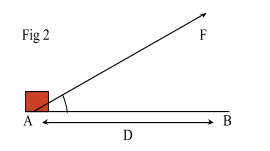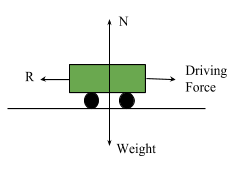# Work, Energy and Power

Contents

## Summary

• Work Done = FD cos ($\theta$) where F is the Force applied to an object, D is the distance moved by the object and$\theta$  is the angle at which the force is applied.
• The energy of a body is its capacity for doing work.
i)$Kinetic\quad Energy\quad =\quad \frac { 1 }{ 2 } \quad m{ v }^{ 2 }$
ii)$Potential\quad Energy\quad =\quad m\quad g\quad h$
• Power is the rate of doing work or the work done per second. The unit of power is the watt.$Power\quad =\quad Force\quad \times \quad Velocity$

#### Work

When a force is applied to an object and the object covers a certain distance in the direction of the force, then the force is said to do work. The unit of work is called Joules (J). One Joule is defined as the work done by a force of 1 Newton in moving a body a distance of 1 metre in the direction of the force.

For large amount of work, the unit used is KiloJoule (KJ) which is equivalent to 1000 Joules.

Let’s study a few different cases of work:

i)    Let F Newton be a force applied to a body. The body covers a distance of D metres from point A to point B in the direction of the force as shown in Fig 1, then the work done by the force is equal to$F\quad \times \quad D$.ii)   Let’s suppose a force F Newton acts on a body and moves it from point A to B and F makes an angle$\theta$  with the direction of the displacement D.

As shown in Fig 2 , the resolved part of F along AB = F cos ($\theta$), therefore only F cos ($\theta$) does the work.

The work done by the force F is$=\quad (F\quad cos(\theta ))\quad \times \quad D$$Work\quad Done\quad =\quad FD\quad cos(\theta )$iii)   If$\theta$  = 90°, i.e the force and the displacements are perpendicular to each other, then the work done by the force is ZERO.

Conversely if the work done by a force F is 0, then F and the displacement are perpendicular to each other.

iv)   If there are different forces, ie p, q, r etc acting on a body, then the work done by these forces in any displacement of the object is equal to the algebraic sum of the work done by p, q , r separately.

#### Example #1

Q. Leo applies a force of 200 N to a box to move it 5 m forward. The Force applied is inclined upwards by 20°. Find the work done.

Solution:

We know:$Work\quad Done\quad =\quad Force\quad \times \quad Distance$

As the force applied has an angle the formula will have cos ($\theta$)$Work\quad Done\quad =\quad FD\quad cos(\theta )$$\theta \quad =\quad 20$°

F = 200 N

D = 5 m

Work Done = 200(5) (cos (20))$Work\quad Done\quad =\quad 1000\quad \times \quad 0.939$

Work Done 939.7 Joules     Ans

#### Energy

The energy of a body is its capacity for doing work. The unit of work is Joule. Since energy of a body is measured by the work, therefore unit of energy is also Joule. There are many kinds of energy but in Dynamics, we are concerned with Mechanical energy which deals with kinetic and potential energy only.

#### Kinetic Energy

Kinetic Energy of a body is measured by the amount of work it does in bringing it from rest to state where its speed is v m/s.

For a body with mass m moving with speed v m/s , the kinetic energy is defined as:$Kinetic\quad Energy\quad =\quad \frac { 1 }{ 2 } \quad m{ v }^{ 2 }$

#### Potential Energy

The potential energy of an object is the energy due to its position with respect to some standard position. The potential energy of an object of mass m at height h above the surface is the work that the object can do in falling to the surface and this is equal to the work done in raising it to the height h.$Potential\quad Energy\quad =\quad m\quad g\quad h$

Lastly , we can recall from GCSE’s, law of conservation of energy which states that energy can be transformed from one form to another but it cannot be created or destroyed.

In a case where kinetic energy and potential energy are increasing or decreasing simultaneously then the formula for word done becomes:

Work done by an external Force = Change in PE + Change in KE + Work Done against Friction

#### Example #2

Q. A load of mass 100 Kg is raised vertically by a crane. During the motion the load passes through the points A and B where B is 1.7 m above A. Its speed at A is 3 m/s and at B is 2 m/s. For the motion from A to B, find:

i) The gain in Gravitational Potential Energy
ii) The loss in Kinetic Energy of the load
iii) The work done by the crane, on the load.

Solution:

We can say that A is the initial point

i) Gain in Gravitational Potential Energy = m g h$=\quad 100\quad \times \quad 10\quad \times \quad 1.7\quad =\quad 1700$ Joules

ii) Loss in Kinetic Energy is going from A to B$=\quad \frac { 1 }{ 2 } \quad m{ v }^{ 2 }$$=\quad \frac { 1 }{ 2 } \quad \times \quad m{ ({ 3 }^{ 2 }\quad -\quad { 2 }^{ 2 }) }\quad =\quad 250$ Joules

iii) Work Done by the crane = Gain in Potential Energy(PE) – Loss in Kinetic Energy(KE)

= 1700 – 250
= 1450 Joules

#### Power

Power is the rate of doing work or the work done per second. The amount of work done per second, therefore indicates how powerful a machine is. This gives us the concept of power or the rate of doing work.

The unit of power is the ”watt”. It is 1 Joule/second and is written as 1W.$Power\quad =\quad Force\quad \times \quad Velocity$

#### Example #3

Q. A train has a maximum speed of 90 Km/h on the level against a resistance of 60,000 N. find the maximum power of the engine.Solution:

As it is mentioned that the train is moving at a maximum speed hence we know that acceleration = 0.

Therefore:

Driving Force = Resistance

F = 60,000 N$Power\quad =\quad Force\quad \times \quad Velocity$$=\quad 60000\quad \times \quad \frac { 90\quad \quad \times \quad 1000 }{ 60\quad \times \quad 60\quad }$

= 1500,000 Watt

= 1500 KW